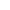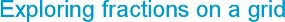###Monday, 18 May 2020
The topic of fractions can be one of the most challenging to teach and learn in primary schools with many hidden misconceptions ready to take hold as children dig deeper into the idea of rational numbers. It is a topic that children need to have time to explore and think about, using a variety of representations to help make sense of it. Here is a simple starting point that can be used in Y4-6 as an activity for exploring fractions as quotients, improper fractions, mixed numbers and equivalence.

This activity is apropriate for children who have already had plenty of experience of using models and images to make sense of fractions. This is abstract in layout, but great for exploring patterns of numbers with fraction notation.

Give each child a copy of the grid below and explain how it is to be completed, with the denominators for each fraction shown on the x-axis and the numerators on the y-axis. Show some examples to help them understand it.### Denominators and numerators

Talk about these two features of numbers written in fractional form. The denominator is the number that shows the units making up the whole, while the number showing the parts of the whole is the numerator.

Make sure they understand that fractions can be interpreted as a quotient, for example 3/4 can show a division: 3 divided by 4. This could be linked to decimal notation if appropriate.

Ask the children to start by completing the squares that are equivalent to 1 (1/1, 2/2, 3/3…). Ask them what they notice. They can then find the fractions equivalent to 1/2, 1/3, 1/4…

They then complete the rest of the grid and look for any patterns. What do they notice on the grid?

### Improper fractions and mixed numbers

Talk about the improper fractions on the grid. Which fractions are greater than 2, 3, 4…?

Ask them to choose one of the improper fractions and convert it to a mixed number. They then show this using a representation of their own choice (cubes, rods, number lines etc). They explain how they converted it.

Children use a counter to cover up numbers you say: Cover up the fraction that is the same as
2 1/3, 1 3/5 etc.

### Equivalent fractions

Children look for pairs of equivalent fractions on the grid. Write a pair, such as 3/4 and 6/8 on the board and ask the class how they can prove that they are equivalent. A rectangle representation of each is particularly helpful for this.

### Ordering fractions

Ask the children to pick any three fractions on the grid. Which has the highest value? Which is the smallest fraction? How do you know? (a washing line as a number line is useful for this). Now put them in order. Repeat this for groups of 3, 4 or 5 fractions.

### Calculating with fractions

Children find pairs of fractions that total 1 on the grid. Display the pairs and ask the children what they notice. Most of the fractions will have the same denominator with the numerators totalling the denominator. Can they find any that don’t follow this rule? For example,
2/6 + 2/3 = 1. Talk about equivalent fractions and use cubes or rods to model this.

### Sorting fractions

This grid makes a good on-going display of fractions that can be used for ‘cover-up’ type games. An interesting task for children to do is to colour-code it in any way they wish, with a key to explain the colour range. They could colour equivalent fractions, fractions of different values or simply those greater than 1 and those less than 1. They choose their own criteria.

Learning objectives that could be targetted through these activities:

Year 4

• Identify pairs of fractions that total 1
• Use diagrams to identify equivalent fractions
• Identify, name and write equivalent fractions of a given fraction
• Interpret mixed numbers and position them on a number line
• Add and subtract fractions with the same denominator

Year 5

• Find and explore patterns of equivalent fractions
• Use equivalent fractions to compare and order fractions whose denominators are all multiples of the same number
• Recognise mixed numbers and improper fractions and convert from one form to the other

Year 6

• Use equivalent fractions to compare and order fractions
• Recall and use equivalences between fractions, decimals and percentages
• Recognise mixed numbers and improper fractions and convert from one form to the other
• Use common factors to simplify fractions and common multiples to show equivalent fractions
• Compare and order fractions, including fractions greater than 1, by converting them to fractions with a common denominator
• Associate a fraction with division to calculate decimal fraction equivalents for a simple fraction
Website design by SiteBuilder Bespoke